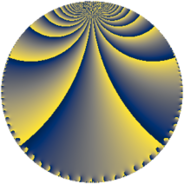# Properties

 Label 105.4.jLevel $105$ Weight $4$ Character orbit 105.j Rep. character $\chi_{105}(8,\cdot)$ Character field $\Q(\zeta_{4})$ Dimension $72$ Newform subspaces $1$ Sturm bound $64$ Trace bound $0$

# Related objects

## Defining parameters

 Level: $$N$$ $$=$$ $$105 = 3 \cdot 5 \cdot 7$$ Weight: $$k$$ $$=$$ $$4$$ Character orbit: $$[\chi]$$ $$=$$ 105.j (of order $$4$$ and degree $$2$$) Character conductor: $$\operatorname{cond}(\chi)$$ $$=$$ $$15$$ Character field: $$\Q(i)$$ Newform subspaces: $$1$$ Sturm bound: $$64$$ Trace bound: $$0$$

## Dimensions

The following table gives the dimensions of various subspaces of $$M_{4}(105, [\chi])$$.

Total New Old
Modular forms 104 72 32
Cusp forms 88 72 16
Eisenstein series 16 0 16

## Trace form

 $$72q + 8q^{3} + O(q^{10})$$ $$72q + 8q^{3} + 144q^{10} - 128q^{12} - 144q^{13} - 16q^{15} - 1608q^{16} + 460q^{18} + 112q^{21} + 576q^{22} + 504q^{25} - 592q^{27} - 580q^{30} - 960q^{31} - 56q^{33} + 928q^{36} + 2088q^{37} + 144q^{40} - 140q^{42} + 240q^{43} - 880q^{45} + 528q^{46} + 3208q^{48} + 1960q^{51} + 240q^{52} + 1200q^{55} - 1112q^{57} + 840q^{58} - 1528q^{60} - 1824q^{61} - 1064q^{63} - 1408q^{66} - 2832q^{67} - 1008q^{70} - 296q^{72} + 1776q^{73} + 5280q^{75} + 7296q^{76} - 4500q^{78} - 4064q^{81} + 1680q^{82} - 10536q^{85} - 392q^{87} - 5352q^{88} - 5664q^{90} + 1008q^{91} - 5488q^{93} - 288q^{96} - 7872q^{97} + O(q^{100})$$

## Decomposition of $$S_{4}^{\mathrm{new}}(105, [\chi])$$ into newform subspaces

Label Dim. $$A$$ Field CM Traces $q$-expansion
$$a_2$$ $$a_3$$ $$a_5$$ $$a_7$$
105.4.j.a $$72$$ $$6.195$$ None $$0$$ $$8$$ $$0$$ $$0$$

## Decomposition of $$S_{4}^{\mathrm{old}}(105, [\chi])$$ into lower level spaces

$$S_{4}^{\mathrm{old}}(105, [\chi]) \cong$$ $$S_{4}^{\mathrm{new}}(15, [\chi])$$$$^{\oplus 2}$$